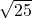## A rectangle has area of 150 in2. The ratio of the length to the width is 3:2. Find the length and width of the rectangle. (This diagra

Question

A rectangle has area of 150 in2. The ratio of the length to the width is 3:2.
Find the length and width of the rectangle. (This diagram is not to scale),

The diagram is just an rectangle, there are no measurements of what the base or width are

in progress 0
2 months 2021-07-26T06:01:54+00:00 2 Answers 0 views 0

1. I’m doing this to but I have no clue how to do this

length = 15 in

width = 10 in

Step-by-step explanation:

let ‘3x’ = length

let ‘2x’ = width

A = l x w

150 = 3x(2x)

150 = 6x²

x² = 150/6

x² = 25

x =x = 5

Length = 3(5) = 15 in

Width = 2(5) = 10 in